...the official site for results from the National Assessment of Educational ProgressHome## Mathematics Sample QuestionsThree sample mathematics questions are presented in this section for each age. The response options for multiple-choice questions are provided as the students saw them, and the oval for the correct answer is filled in. All constructed-response questions in the long-term trend mathematics assessment were scored as correct or
incorrect, and the correct response is shown on the answer line.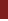Age 9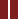Age 13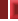Age 17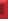Sample Question 1Sample Question 2Sample Question 3Sample question 3 required students to solve a multi-step problem involving the perimeter and area of a square.

 59 percent of 17-year-olds answered this question correctly.

The perimeter of a square is 36 centimeters. What is the area of the square?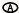6 square cm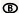9 square cm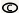18 square cm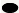81 square cm